# Quadratic Function y ax 2 bx c Quadratic

• Slides: 7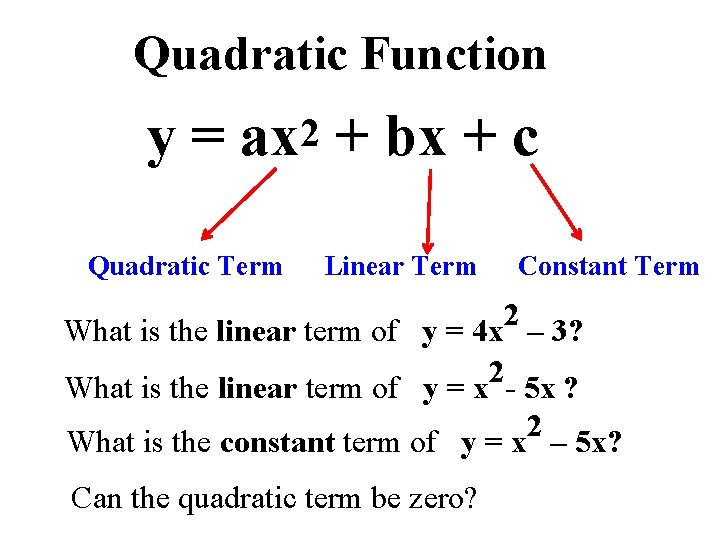Quadratic Function y = ax 2 + bx + c Quadratic Term Linear Term Constant Term 2 What is the linear term of y = 4 x – 3? 0 x 2 What is the linear term of y = x - 5 x ? -5 x What is the constant term of y = x 2 – 5 x? 0 Can the quadratic term be zero? No!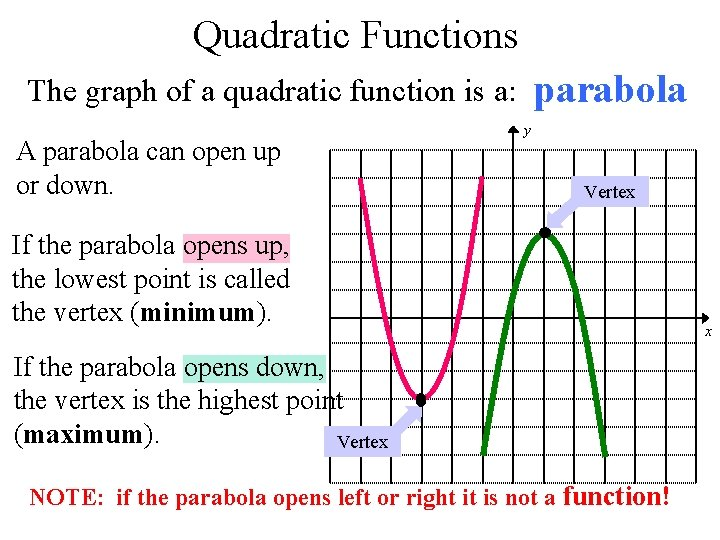Quadratic Functions parabola The graph of a quadratic function is a: A parabola can open up or down. y Vertex If the parabola opens up, the lowest point is called the vertex (minimum). If the parabola opens down, the vertex is the highest point (maximum). Vertex NOTE: if the parabola opens left or right it is not a function! x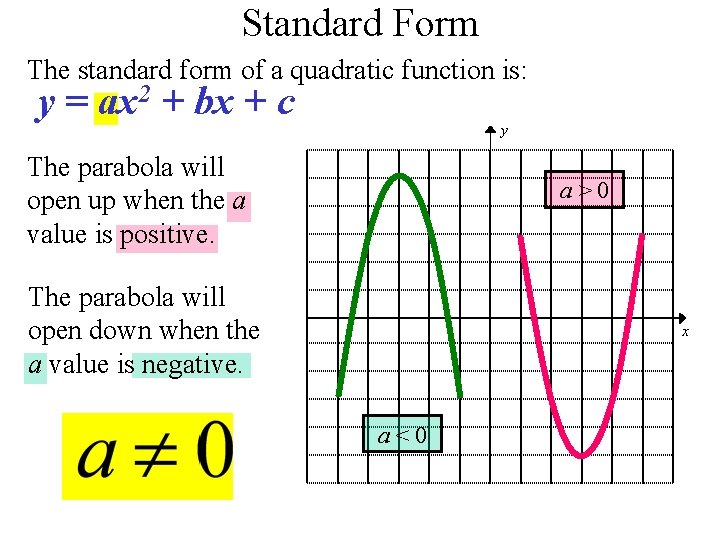Standard Form The standard form of a quadratic function is: y = ax 2 + bx + c y The parabola will open up when the a value is positive. a>0 The parabola will open down when the a value is negative. x a<0Axis of Symmetry Parabolas are symmetric. If we drew a line down the middle of the parabola, we could fold the parabola in half. Axisy of Symmetry We call this line the Axis of symmetry. x If we graph one side of the parabola, we could REFLECT it over the Axis of symmetry to graph the other side. The Axis of symmetry ALWAYS passes through the vertex.Finding the Axis of Symmetry When a quadratic function is in standard form y = ax 2 + bx + c, the equation of the Axis of symmetry is This is best read as … ‘the opposite of b divided by the quantity of 2 times a. ’ Find the Axis of symmetry for y = 3 x 2 – 18 x + 7 a = 3 b = -18 The Axis of symmetry is x=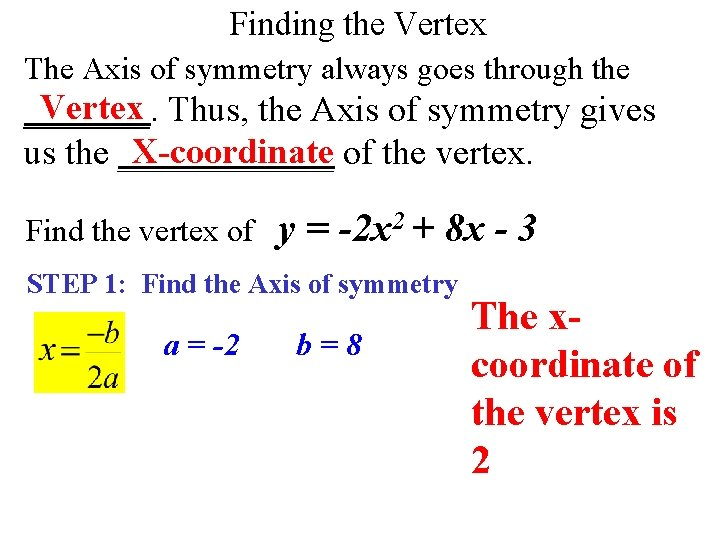Finding the Vertex The Axis of symmetry always goes through the Vertex Thus, the Axis of symmetry gives _______. X-coordinate of the vertex. us the ______ Find the vertex of y = -2 x 2 + 8 x - 3 STEP 1: Find the Axis of symmetry a = -2 b=8 The xcoordinate of the vertex is 2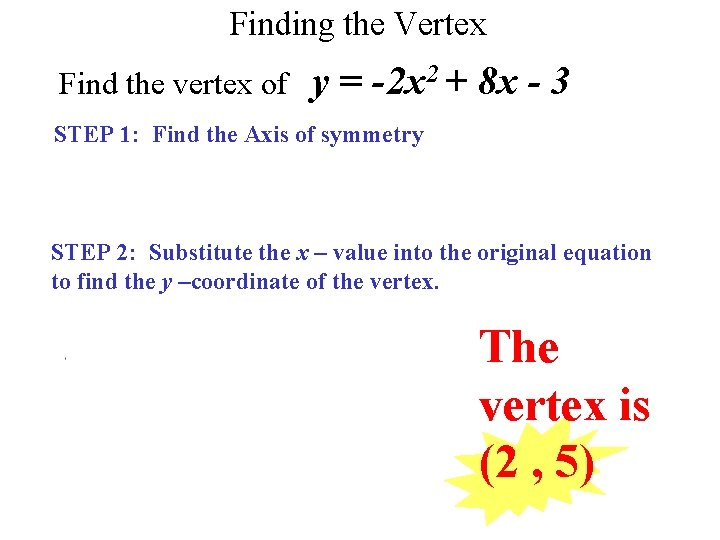Finding the Vertex Find the vertex of y = -2 x 2 + 8 x - 3 STEP 1: Find the Axis of symmetry STEP 2: Substitute the x – value into the original equation to find the y –coordinate of the vertex. The vertex is (2 , 5)